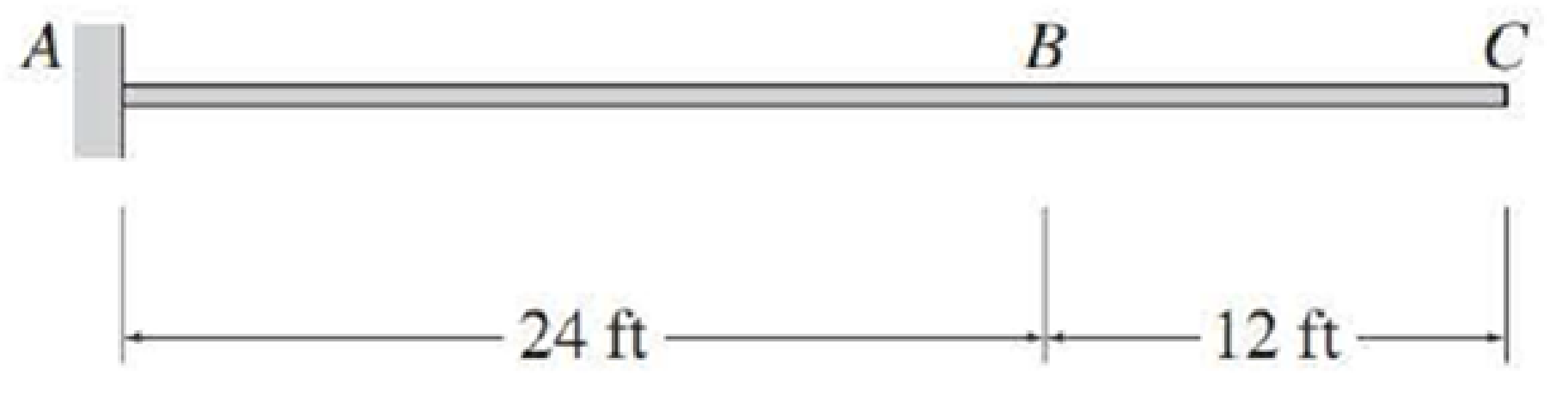# For the cantilever beam of Problem 8.5, determine the maximum upward vertical reaction and the maximum counterclockwise reaction moment at support A due to a concentrated live load of 25 k, a uniformly distributed live load of 2 k/ft, and a uniformly distributed dead load of 0.5 k/ft. FIG. P8.5, P8.58

#### Solutions

Chapter
Section
Chapter 9, Problem 5P
Textbook Problem
16 views

## For the cantilever beam of Problem 8.5, determine the maximum upward vertical reaction and the maximum counterclockwise reaction moment at support A due to a concentrated live load of 25 k, a uniformly distributed live load of 2 k/ft, and a uniformly distributed dead load of 0.5 k/ft.FIG. P8.5, P8.58

To determine

Find the maximum upward vertical reaction and the maximum counterclockwise reaction moment at support A.

### Explanation of Solution

Given Information:

The concentrated live load (P) is 25 k.

The uniformly distributed live load (wL) is 2 k/ft.

Calculation:

Sketch the free body diagram of beam as shown in Figure 1.

Apply a 1 k unit moving load at a distance of x from left end A.

Refer Figure 1.

Find the equation of support reaction (Ay) at A using equilibrium equation:

Consider upward force as positive (+) and downward force as negative ().

ΣFy=0Ay1=0Ay=1k

The value of influence line ordinate of reaction is 1 k/k applied throughout the length of the beam.

Draw the influence line diagram for the vertical reaction at support A as shown in Figure 2.

Refer Figure 1.

The maximum influence line ordinate due to upward reaction at A is 1 k/k.

Find the area of the influence line diagram (A) for the vertical reaction at support A.

A=L(1)

Here, L is the length of the beam.

Substitute 36 ft for L.

A=(36)(1)A=36ft

Find the maximum upward vertical reaction at support A using the equation.

MaximumupwardAy=[P(maximuminfluencelineordinate)+wL(A)+wD(A)]

Substitute 25 k for P, 1 k/k for maximuminfluencelineordinate, 2 k/ft for wL, 36 ft for A, and 0.5 k/ft for wD.

MaximumupwardAy=25(1)+2(36)+0.5(36)=115k()

Therefore, the maximum upward vertical reaction at support A is 115k_.

Find the equation of reaction moment (MA) at support A.

Consider moment equilibrium at point A.

Consider clockwise moment as negative and anticlockwise moment as positive.

Sum of moment at point A is zero.

ΣMA=0MA1(x)=0MA=x        (1)

Consider Equation (1)

### Still sussing out bartleby?

Check out a sample textbook solution.

See a sample solution

#### The Solution to Your Study Problems

Bartleby provides explanations to thousands of textbook problems written by our experts, many with advanced degrees!

Get Started

Find more solutions based on key concepts
When learning to play some sports, such as tennis, golf, or baseball, often you are told to follow through with...

Engineering Fundamentals: An Introduction to Engineering (MindTap Course List)

What e input masksâ€� What are validation rules? Why are they important?

Systems Analysis and Design (Shelly Cashman Series) (MindTap Course List)

_____ measures the amount of time it takes a storage device to locate an item on a storage medium and/or the ti...

Enhanced Discovering Computers 2017 (Shelly Cashman Series) (MindTap Course List)

Calculate the reaction at D for the structure described in Prob. 5.11.

International Edition---engineering Mechanics: Statics, 4th Edition

How is a CBN wheel trued and dressed?

Precision Machining Technology (MindTap Course List)

What does milky colored ATF indicate?

Automotive Technology: A Systems Approach (MindTap Course List)

Convert P = 5.00 atm into Pa, bar, and psia.

Fundamentals of Chemical Engineering Thermodynamics (MindTap Course List)

Name two types of respirators and describe how they work.

Welding: Principles and Applications (MindTap Course List)

If your motherboard supports ECC DDR3 memory, can you substitute non-ECC DDR3 memory?

A+ Guide to Hardware (Standalone Book) (MindTap Course List)